## Monday, April 7, 2014

### Calculating First and Last Non-Empty in Tableau

Today, we will talk about calculating the first and last non-empty values in Tableau.  These calculations are extremely useful when you are looking at values that may be missing for the current time period.  For this demonstration, we will be using a mock-up data set designed for this demonstration.

First, let's look at the data.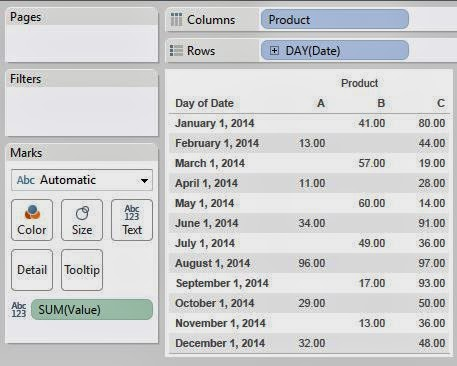Data
As you can see, products A and B are missing values for certain days while product C has all values.  Now, let's say that we want to know what the value was for these products in September. Well, we can look at September and see that Product A has no value.  Then, we can easily scroll our eyes up to August and see that Product A had a value of 96.  But, how do we get Tableau to do this work for us?

Well, let's start by looking at how to calculate the first non-empty value.  For instance, this value would be 41 and 80 for Products B and C because they have values for January.  However, Product A has no value for January.  Therefore, the value for Product A would be 13.  Let's look at how to get Tableau to tell us this.

The first thing we need to do is find the indices for all of the non-empty cells.  We can do this using the following calculation:Non-Empty IndexValue and Non-Empty Index by Month and Product
As you can see, this calculation only returns the row index for non-empty values.  Now, if we want to find the first non-empty value, then we need to find the value corresponding to the smallest non-empty index.First Non-Empty IndexValue, Non-Empty Index, and First Non-Empty Index by Month and Product
Voila!  We now know what index references the first non-empty value.  Now, we just need to use the LOOKUP() function to return that value.First Non-Empty ValueValue and First Non-Empty Value by Month and Product
We can see that we have now returned the first non-empty value in the column.  However, this was not quite our original problem.  We wanted to be able to return the most recent value in the column.  In some circles, this is known as the "Last Non-Empty".  So, what would happen if we changed our calculation to return the max of the indexes instead of the min?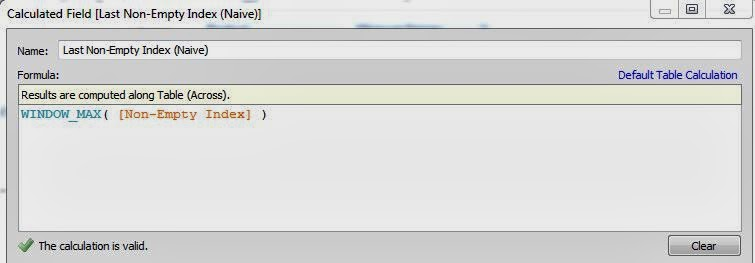Last Non-Empty Index (Naive)Value and Last Non-Empty Index by Month and Product (Naive)
Well, this just returns the last non-empty index with no consideration of the current position in the table.  However, we can alter the calculation slightly to return the last non-empty index without looking past the current row.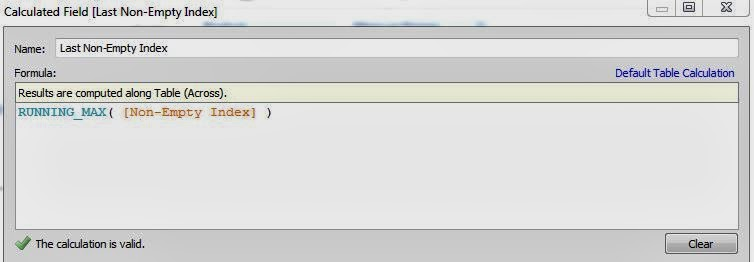Last Non-Empty IndexValue and Last Non-Empty Index by Month and Product
Now, let's use this calculation to find the corresponding values.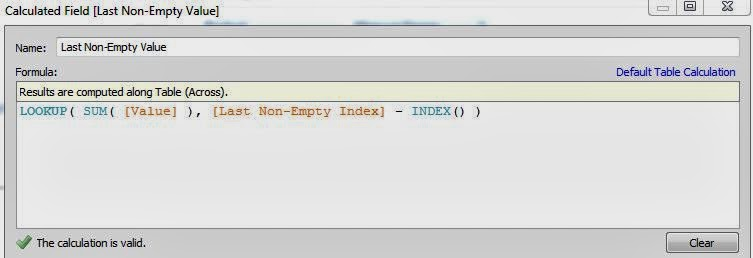Last Non-Empty ValueValue and Last Non-Empty Value by Month and Product
As you can see, this procedure is not overly difficult.  All it requires is a little ingenuity and a basic understanding of table calculations.  The cool thing about this technique is that it's extremely useful for so many different applications.  We've had plenty of clients put a lot of effort into find the last selling price for an item.  There's even more to do with this as well.  This procedure could easily be augmented to look at the last selling price for one item OR the current selling price for another item.  Thanks for reading.  We hope you found this informative.

Edit: Jim Wahl correctly asserted that you can utilize the PREVIOUS_VALUE() function to achieve similar results.  Just goes to show that there's typically more than one path to the right answer.

Data Analytics Consultant
Mariner, LLC
llewellyn.wb@gmail.com

1.Great explanation! I've been searching for this type of solution for a long time (it should be a standard feature, though). I'm looking forward to test it.

- Francisco

2.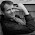Another approach, at least to the specific example above, is to use Tableau's PREVIOUS_VALUE() function.

SUM Value =
IFNULL(SUM(Value), PREVIOUS_VALUE(0))

WHEN SUM(Value) is null, PREVIOUS_VALUE() will use the value in the previous row.

When the first value is NULL, as in Product A, you'll get 0---the argument to PREVIOUS_VALUE---but you could avoid this by checking for the first row and using the regular aggregate SUM(Value)
IF FIRST() == 0 THEN SUM(Value)
ELSE ...
END

1.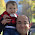Good eye sir! I never even considered using PREVIOUS_VALUE(). Thanks for the feedback.

3.Thanks brad that was really good
Hi jim, could you be more specific about the use of PREVIOUS_VALUE in the calculated fields. screen shots would be much more useful to understand

4.THANK YOU! I had been having trouble with a similar problem for a week. I appreciate the step-by-step guide.

5.Realmente é às vezes essencial para expor bem para fácil
de prestar atenção, como vi aqui neste texto. Irei procurar
outro texto do seu blog.